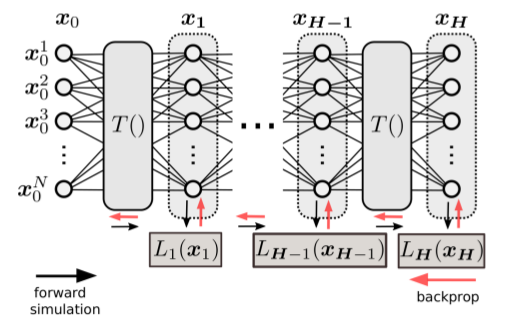# Custom implementation FC DNN, help needed with applying torch.optim

Hello everyone!

i am trying to implement custom DNN, approx stucture can be seen at next imageThe idea behind is that there is only one learnable parameter here, and it is initial state X (input tensor), all other `params` are fixed (not learnable).

We managed to make it work using custom nn.Module class with calculating gradients and manually applying them

``````class Linear(nn.Module):
def __init__(self):                     #input_features, output_features, bias=True):
super(Linear, self).__init__()

def forward(self, input):
state, cost = input

rf, af = calc_forces(state, goals, param.pedestrians_speed, param.k, param.alpha, param.ped_radius, param.ped_mass, param.betta)
F = rf + af
out = pose_propagation(F, state, param.DT, param.pedestrians_speed)
temp = calc_cost_function(param.a, param.b, param.e, goals, robot_init_pose, out, observed_state)
cost = cost + temp.view(-1,1)
return (out, cost)

....
modules = []
for i in range (0, number_of_layers):
modules.append(Linear())

sequential = nn.Sequential(*modules)
....
inner_data, cost = sequential((inner_data, cost))
cost = cost.sum()
cost.backward()

``````

so, question is next:
May someone, point me in the right direction, how to apply torch.optim.SGD or any similar in such case?
The problem is that usually they operates with nn.Parameters, in mine case it is not clear how to introduce these parameter, because these parameter became defined only at first iteration.

i’ve tried to use

``````class Linear(nn.Module):
def __init__(self):                     #input_features, output_features, bias=True):
super(Linear, self).__init__()
self.register_parameter('init_state', None)

def forward(self, input):
state, cost = input

if self.init_state is None:
rf, af = calc_forces(self.init_state, goals, param.pedestrians_speed, param.k, param.alpha, param.ped_radius, param.ped_mass, param.betta)
F = rf + af
out = pose_propagation(F, self.init_state, param.DT, param.pedestrians_speed)
else:
if type(state) == type(nn.Parameter(torch.Tensor(1))):
state = torch.tensor(state)
rf, af = calc_forces(state, goals, param.pedestrians_speed, param.k, param.alpha, param.ped_radius, param.ped_mass, param.betta)
F = rf + af
out = pose_propagation(F, state, param.DT, param.pedestrians_speed)

temp = calc_cost_function(param.a, param.b, param.e, goals, robot_init_pose, out, observed_state)
cost = cost + temp.view(-1,1)
return (out, cost)
``````

but it doesn`t helped much (sequential.init_state.grad always None)